### Home > CALC > Chapter Ch12 > Lesson 12.2.2 > Problem12-78

12-78.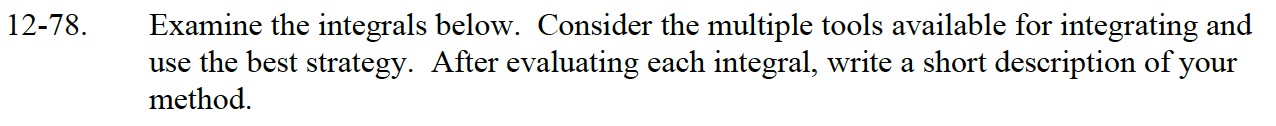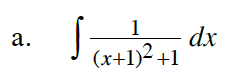Let u = x + 1.
Once you rewrite the integrand, you should recognize it as a special case.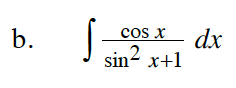Let u = sin(x).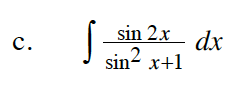sin(2x) = 2sin(x)cos(x)

Let u = sin2(x) + 1.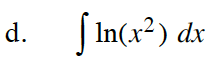ln(x2) = 2ln(x)